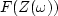Next: Dividing by zero smoothly Up: Univariate problems Previous: References

# HOW TO DIVIDE NOISY SIGNALS

Another univariate statistical problem arises in Fourier analysis, where we seek a best answer'' at each frequency, then combine all the frequencies to get a best signal. Thus emerges a wide family of interesting and useful applications. However, Fourier analysis first requires us to introduce complex numbers into statistical estimation.

Multiplication in the Fourier domain is convolution in the time domain. Fourier-domain division is time-domain deconvolution. In chapterwe encountered the polynomial-division feedback operation X(Z)=Y(Z)/F(Z). This division is challenging when F has observational error. By switching from the Z-domain to the-domain we avoid needing to know if F is minimum phase. The-domain has pitfalls too, however. We may find for some realthatvanishes, so we cannot divide by that F. Failure erupts if zero division occurs. More insidious are the poor results we obtain when zero division is avoided by a near miss.Next: Dividing by zero smoothly Up: Univariate problems Previous: References
Stanford Exploration Project
10/21/1998